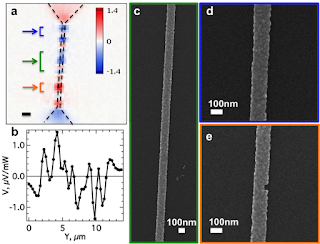## Sunday, July 16, 2017

### A thermoelectric surprise in metals

Earlier this year I'd described what thermoelectricity is, and I'd also discussed recent work of ours where we used a laser as a scan-able heat source, and were then able to see nicely the fact that changing the size of a nanoscale metal structure can vary the material's thermoelectric properties, and make a thermocouple out of a single metal.With this same measurement technique, we found a result that we thought was rather strange and surprising, which we have written up here.   Take a moderately long wire, say 120 nm wide and several microns long, made by patterning a 15 nm thick Au film.  Hook up basically a volt meter to the ends of the wire, and scan the laser spot along the length of the wire, recording the voltage as a function of the laser position.  If the wire is nice and homogeneous, you'd expect not to see to much until you get to the ends of the wire where it widens out into bigger contacts.  (There the size variation should make the skinny/wide junction act like a thermocouple.)   Instead, we see the result shown here in the figure (fig. 2 of the paper).  There is a great deal of spatial variability in the photothermoelectric voltage, like the wire is actually made up of a whole bunch of little thermocouples!

Note that your eye tends to pick out a spatial scale in panel (a) comparable to the 1 micron scale bar.  That's a bit misleading; the spot size of the laser in our system is about 1.8 microns, so this measurement approach would not pick up much smaller spatial scales of variation.

The metal wire is polycrystalline, and if you look at the electron microscope images in panels (c, d, e) you can make out a grain structure with lateral grain sizes of 15-20 nm.  Maybe the wire isn't all that homogeneous?  One standard way physicists look at the quality of metal films is to consider the electrical resistance of a square patch of film ($R_{\square}$, the "sheet resistance" or "resistance per square"), and compare that number with the "resistance quantum", $R_{\mathrm{q}}\equiv h/2e^2$, a combination of fundamental constants that sets a scale for resistance.  If you had two pieces of metal touching at a single atom, the resistance between them would be around the resistance quantum.  For our wire material, $R_{\square}$ is a little under 4 $\Omega$, so $R_{\square} << R_{\mathrm{q}}$, implying that the grains of our material are very well-connected - that it should act like a pretty homogeneous film.  This is why the variation shown in the figure is surprising.  Annealing the wires does change the voltage pattern as well as smoothing it out.  This is a pretty good indicator that the grain boundaries really are important here.  We hope to understand this better - it's always fun when a system thought to be well understood surprises you.

Ted Sanders said...

Nifty. Thank you for writing this up.Anonymous said...

Hi Doug, why do you think the sign of photovoltage changes along the wire?

Douglas Natelson said...

Anon, if there is a spatially varying Seebeck coefficient $S(x)$, and the sample is heated inhomogeneously via the spot from the laser, it's not that hard to have voltage vs. laser position be non-monotonic. One way to see this is to start from the definition of $S$, where locally $\nabla V \equiv -S \nabla T$. Then $V = -\int S(x)~dT(x)/dx~dx$ where the integral runs from one end of the sample to the other. If you integrate by parts, you get $V = -[S(1)T(1)-S(2)T(2)] + \int T(x)~dS/dx~dx$, where 1 and 2 denote evaluation at the ends of the sample. That second integral is the key, because $dS/dx$ can take on either sign as $S$ varies along the sample.

Douglas Natelson said...

(somehow blogger ate the rest of my explanation.) For each position of the laser, we get some profile $T(x)$, and we can compute that integral. If $T(x)$ happens to be largest right where $dS/dx$ is positive, you'd likely end up with a positive number. Conversely, if $T(x)$ is largest where $dS/dx$ happens to be negative, then you could get a negative net voltage. Depending on how the Seebeck coefficient varies along the sample, you could then get sign flips of $V$ as you scan the laser spot along the sample.Anonymous said...

I see, thank you for the explanation! And do you think that the Seebeck coefficient can vary that much along the wire? I.e., does this all work out quantitatively?

Douglas Natelson said...

Anon, yes, it seems so. Variations of around 10% or so along the length of the device would be about right.